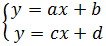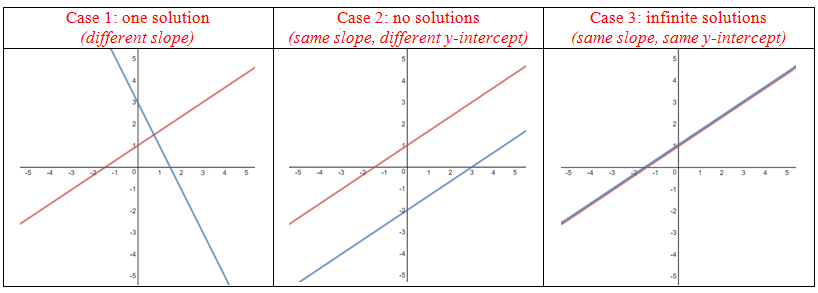# System of linear equations

### System of linear equations

#### Lessons

• Introduction
$\bullet$ The solutions to a system of equations are the points of intersection of the graphs.
$\bullet$ For a system consisting of two linear equations:There are 3 cases to consider:• 1.
Solving by Elimination
Solve each linear system algebraically by elimination.
a)
$3x+4y=11$
$x-4y=-7$

b)
$6x-1=2y$
$9x+4=5y$

c)
$3(x+2)-(y+7)=-10$
$5(x+1)+(y+3)=19$

• 2.
Solving by Substitution
Solve each linear system algebraically by substitution.
a)
$6x-1y=7$
$-9x+2y=7$

b)
$3(x+2)-(y+7)=4$
$5(x+1)+4(y+3)=31$

c)
$x - y = -1$
$3x + 5y = 21$